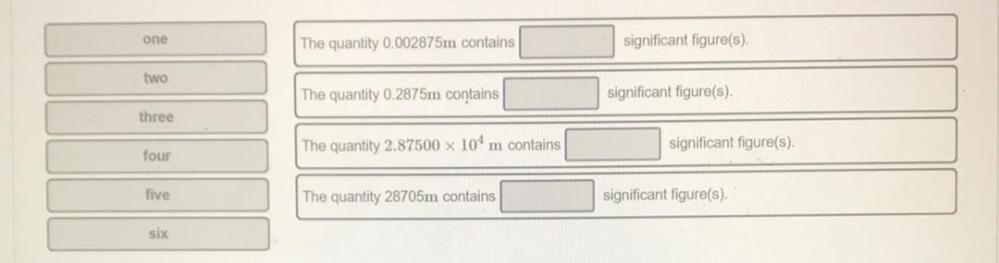Question:

# 1. The quantity 0.002875m contains _______ significant figure(s). 2. The quantity 0.2875m contains _______ significant figure(s) 3. The quantity 2.87500 x 10⁴ m contains _______ significant figure(s).1. The quantity 0.002875m contains _______ significant figure(s). 2. The quantity 0.2875m contains _______ significant figure(s) 3. The quantity 2.87500 x 10⁴ m contains _______ significant figure(s). 4. The quantity 28705m contains _______ significant figure(s)